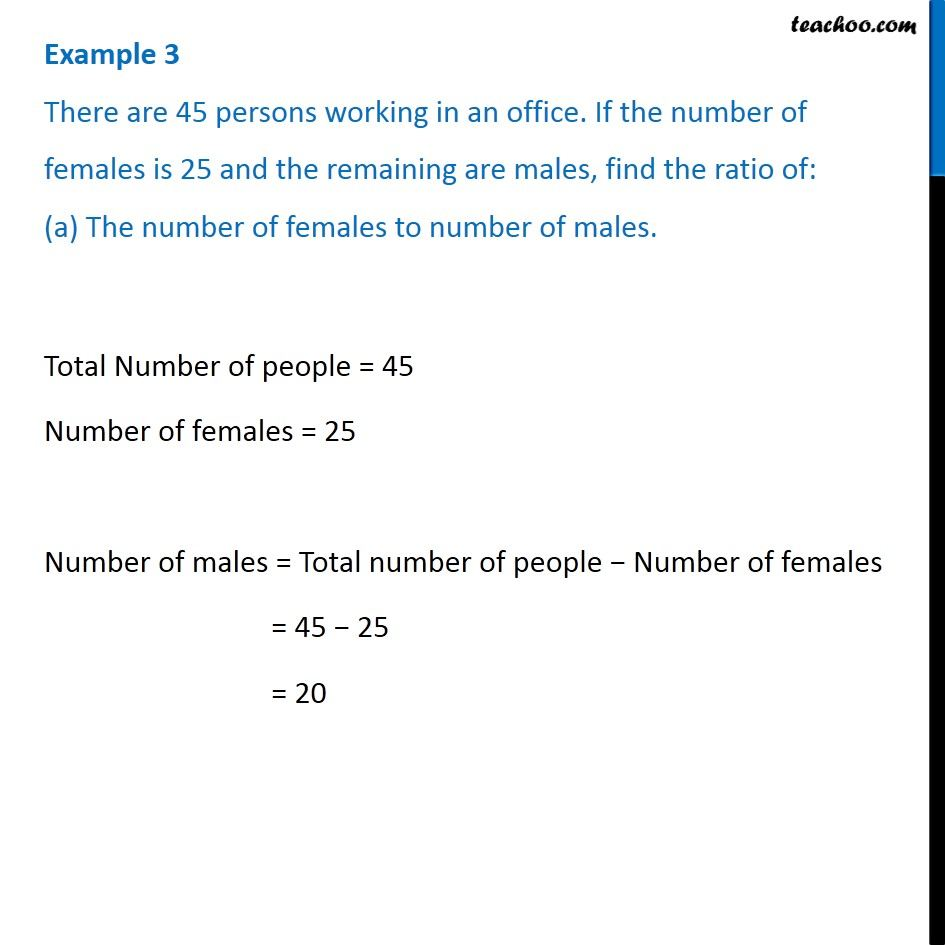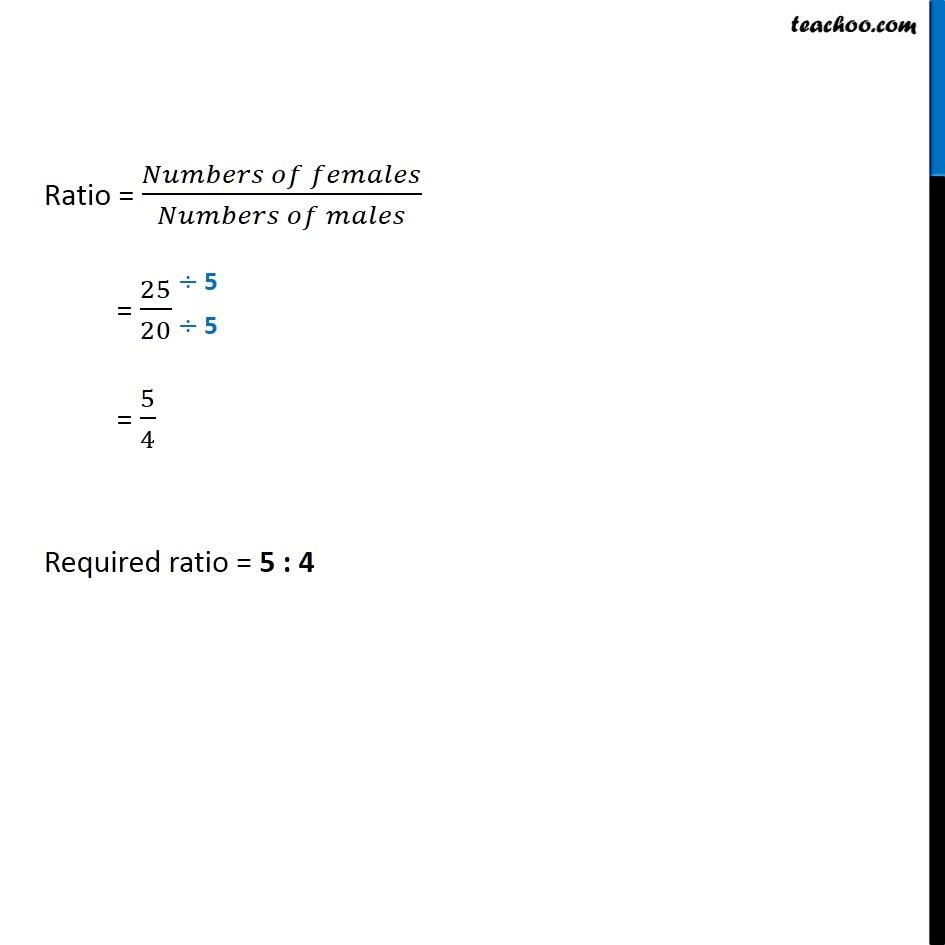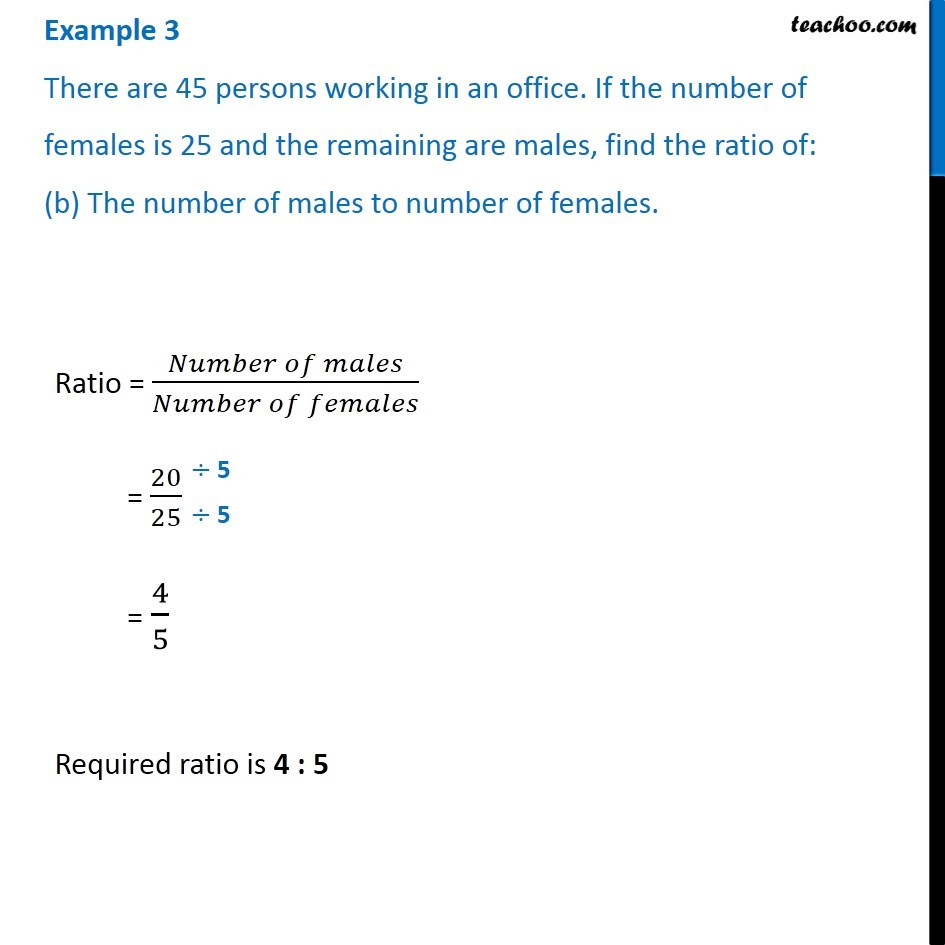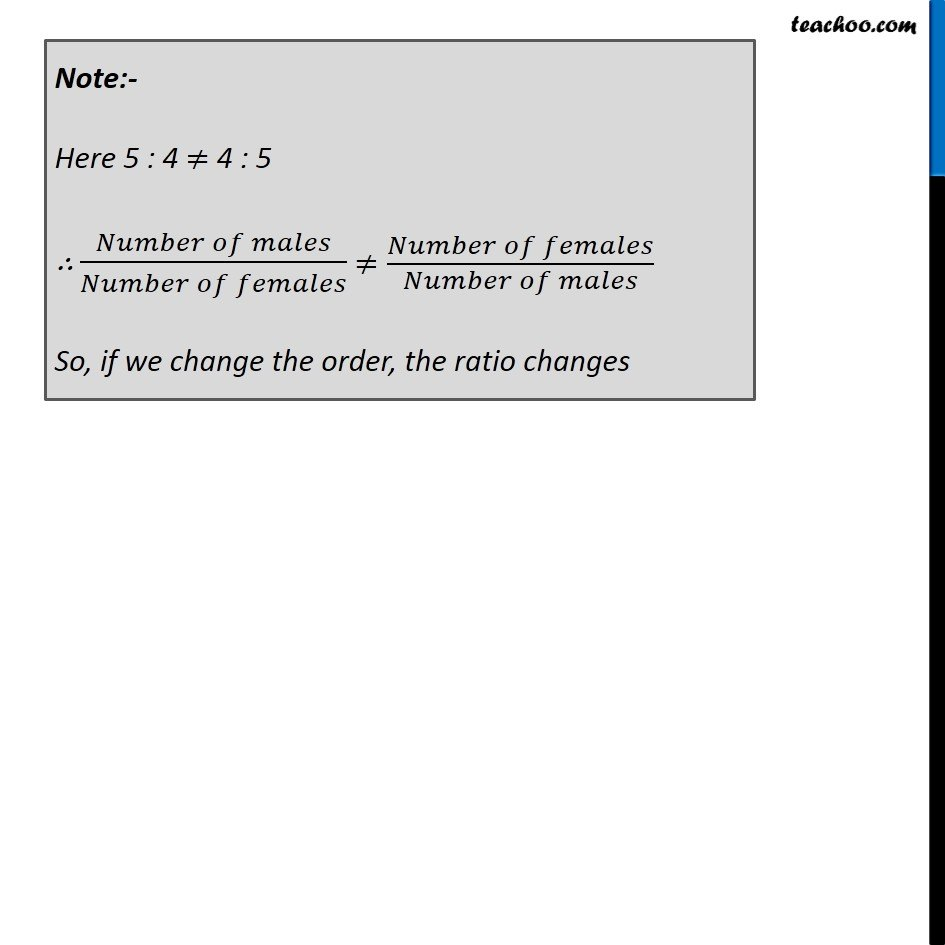Examples

Chapter 12 Class 6 Ratio and Proportion
Serial order wiseLearn in your speed, with individual attention - Teachoo Maths 1-on-1 Class

### Transcript

Example 3 There are 45 persons working in an office. If the number of females is 25 and the remaining are males, find the ratio of: (a) The number of females to number of males. Total Number of people = 45 Number of females = 25 Number of males = Total number of people − Number of females = 45 − 25 = 20 Ratio = (𝑁𝑢𝑚𝑏𝑒𝑟𝑠 𝑜𝑓 𝑓𝑒𝑚𝑎𝑙𝑒𝑠)/(𝑁𝑢𝑚𝑏𝑒𝑟𝑠 𝑜𝑓 𝑚𝑎𝑙𝑒𝑠) = 25/20 = 5/4 Required ratio = 5 : 4 Example 3 There are 45 persons working in an office. If the number of females is 25 and the remaining are males, find the ratio of: (b) The number of males to number of females.Ratio = (𝑁𝑢𝑚𝑏𝑒𝑟 𝑜𝑓 𝑚𝑎𝑙𝑒𝑠)/(𝑁𝑢𝑚𝑏𝑒𝑟 𝑜𝑓 𝑓𝑒𝑚𝑎𝑙𝑒𝑠) = 20/25 = 4/5 Required ratio is 4 : 5 Note:- Here 5 : 4 ≠ 4 : 5 ∴ (𝑁𝑢𝑚𝑏𝑒𝑟 𝑜𝑓 𝑚𝑎𝑙𝑒𝑠)/(𝑁𝑢𝑚𝑏𝑒𝑟 𝑜𝑓 𝑓𝑒𝑚𝑎𝑙𝑒𝑠) ≠(𝑁𝑢𝑚𝑏𝑒𝑟 𝑜𝑓 𝑓𝑒𝑚𝑎𝑙𝑒𝑠)/(𝑁𝑢𝑚𝑏𝑒𝑟 𝑜𝑓 𝑚𝑎𝑙𝑒𝑠) So, if we change the order, the ratio changes